Courses

# Continuous Time Fourier Transformer (CTFT) - 2

## 10 Questions MCQ Test Topicwise Question Bank for Electrical Engineering | Continuous Time Fourier Transformer (CTFT) - 2

Description
This mock test of Continuous Time Fourier Transformer (CTFT) - 2 for Electrical Engineering (EE) helps you for every Electrical Engineering (EE) entrance exam. This contains 10 Multiple Choice Questions for Electrical Engineering (EE) Continuous Time Fourier Transformer (CTFT) - 2 (mcq) to study with solutions a complete question bank. The solved questions answers in this Continuous Time Fourier Transformer (CTFT) - 2 quiz give you a good mix of easy questions and tough questions. Electrical Engineering (EE) students definitely take this Continuous Time Fourier Transformer (CTFT) - 2 exercise for a better result in the exam. You can find other Continuous Time Fourier Transformer (CTFT) - 2 extra questions, long questions & short questions for Electrical Engineering (EE) on EduRev as well by searching above.
QUESTION: 1

### If x(n) = (1/2)n u(n), y(n) = x2(n) and (eiω) be the fourier transform of y(n), then y(e0) is

Solution: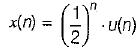and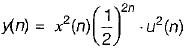⇒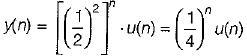then,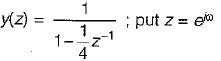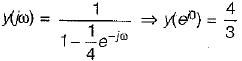QUESTION: 2

### For a signal x(t), the fourier transform is X(f), then inverse fourier transform of x(3f + 2) is given by

Solution: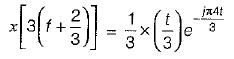Apply scaling and shifting property.

QUESTION: 3

### The Fourier transform of given signal x(t)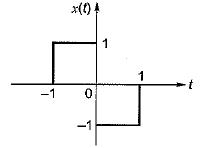Solution: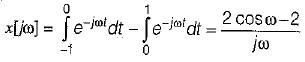QUESTION: 4

Fourier transform of x(t) = eat u (-t), a > 0 is

Solution:
QUESTION: 5

Determine the fourier transform of the signal x(t) shown in figure.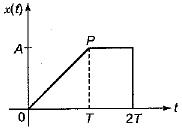Solution: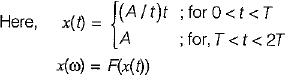⇒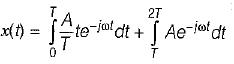⇒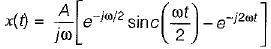QUESTION: 6

Match List-I [Function f(t)] with List-II [Fourier transform F(ω)] and select the correct answer using the codes given below the lists:
List- I
A. f(t – t0)
B. f(t) ejω0t
C. f1(t) . f2(t)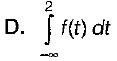List-ll
1. f(ω – ω0)
2.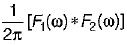3.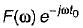4.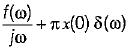5.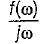Codes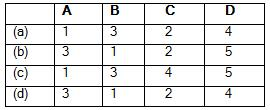Solution:
QUESTION: 7

Consider a continuous time low pass filter whose impulse response h(t) is known to be real and whouse frequency response magnitude is given by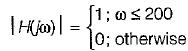Determine the value of h(t) if the group delay function is specified as t(ω) = 5.

Solution:

Since,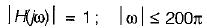0; otherwise
using duality property,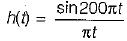Since group delay is constant and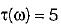So,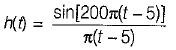QUESTION: 8

Consider a signal x1(t) having a fourier transform x1(jω). An another signal x2(t) having fourier transform x2(jω) is related to x1 (t) by x2(jω) = [1 +sgn(ω)] x1(jω) x2(t) in terms of x1(t) is equal to

Solution:

Since,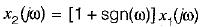or,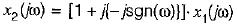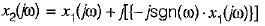or,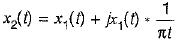because,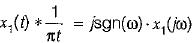using, Hilbert transform

QUESTION: 9

A Fourier transform pair is as follows: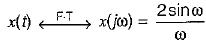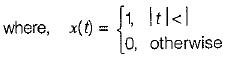The Fourier transform of given signal y(t) is

Solution: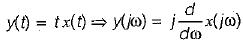⇒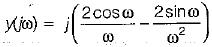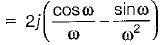QUESTION: 10

Suppose; y(t) = x(t) cost
and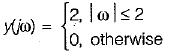then x(t) will be

Solution: